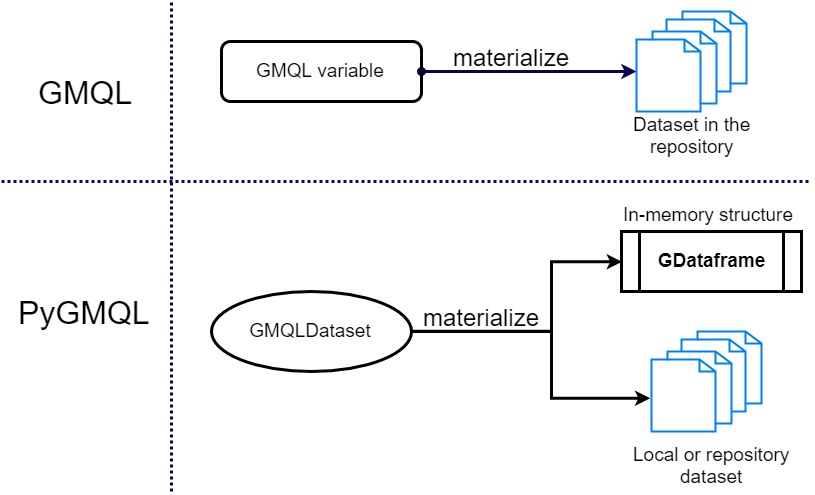# Introduction¶

In this brief tutorial we will explain the typical workflow of the user of PyGMQL. In the github page of the project you can find a lot more example of usage of the library.

You can use this library both interactively and programmatically. We strongly suggest to use it inside a Jupyter notebook for the best graphical render and data exploration.

## Importing the library¶

To import the library simply type:

```import gmql as gl
```

If it is the first time you use PyGMQL, the Scala backend program will be downloaded. Therefore we suggest to be connected to the internet the first time you use the library.

The first thing we want to do with PyGMQL is to load our data. You can do that by calling the `gmql.dataset.loaders.Loader.load_from_path()`. This method loads a reference to a local gmql dataset in memory and creates a `GMQLDataset`. If the dataset in the specified directory is already GMQL standard (has the xml schema file), you only need to do the following:

```dataset = gl.load_from_path(local_path="path/to/local/dataset")
```

while, if the dataset has no schema, you need to provide it manually. This can be done by creating a custom parser using `RegionParser` like in the following:

```custom_parser = gl.parsers.RegionParser(chrPos=0, startPos=1, stopPos=2,
otherPos=[(3, "gene", "string")])
```

## Writing a GMQL query¶

Now we have a dataset. We can now perform some GMQL operations on it. For example, we want to select samples that satisfy a specific metadata condition:

```selected_samples = dataset[ (dataset['cell'] == 'es') | (dataset['tumor'] == 'brca') ]
```

Each operation on a GMQLDataset returns an other GMQLDataset. You can also do operations using two datasets:

```other_dataset = gl.load_from_path("path/to/other/local/dataset")

union_dataset = dataset.union(other_dataset)                            # the union
join_dataset = dataset.join(other_dataset, predicate=[gl.MD(10000)])    # a join
```

## Materializing a result¶

PyGMQL implements a lazy execution strategy. No operation is performed until a materialize operation is requested:

```result = join_dataset.materialize()
```

If nothing is passed to the materialize operation, all the data are directly loaded in memory without writing the result dataset to the disk. If you want also to save the data for future computation you need to specify the output path:

```result = join_dataset.materialize("path/to/output/dataset")
```

## The result data structure¶

When you materialize a result, a `GDataframe` object is returned. This object is a wrapper of two pandas dataframes, one for the regions and one for the metadata. You can access them in the following way:

```result.meta     # for the metadata
result.regs     # for the regions
```

These dataframes are structured as follows:

• The region dataframe puts in every line a different region. The source sample for the specific region is the index of the line. Each column represent a field of the region data.
• The metadata dataframe has one row for each sample in the dataset. Each column represent a different metadata attribute. Each cell of this dataframe represent the values of a specific metadata for that specific sample. Multiple values are allowed for each cell.